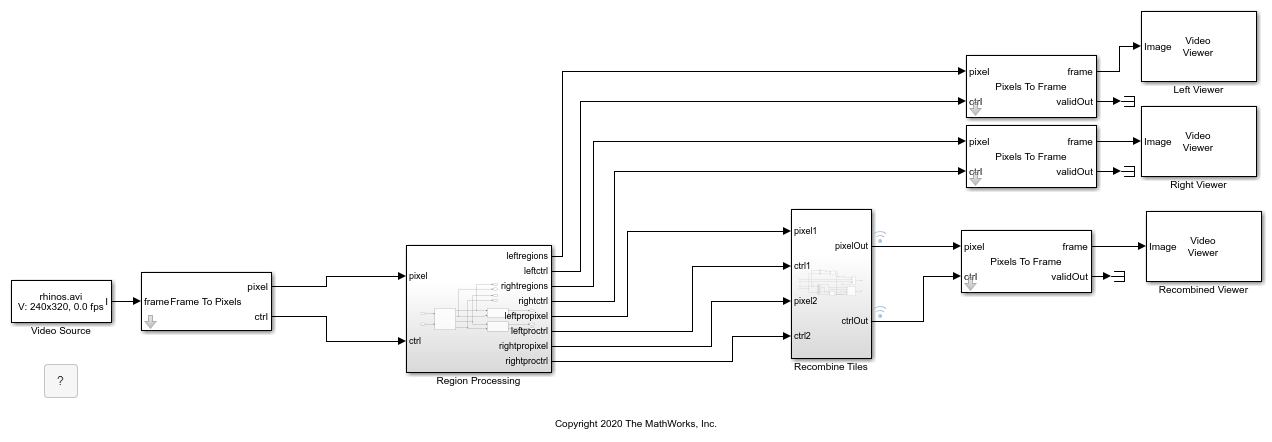# Select Regions for Vertical Reuse

This example shows how to divide a frame into tiled regions of interest (ROIs) and use those regions to configure the ROI Selector block for vertical reuse.

Vertical reuse means dividing each frame into vertically-aligned regions where each column of regions shares a pixel stream. This arrangement enables parallel processing of each column, and the reuse of downstream processing logic for each region in the column.

Set up the size of the frame.

```frmActiveLines = 240; frmActivePixels = 320; ```

Divide the frame into equally-sized vertically-aligned regions, or tiles. The `visionhdlframetoregions` function returns an array of such regions, where each region is defined by four coordinates, and is of the form [ hPos vPos hSize vSize ]. These tile counts divide evenly into the frame dimensions, so no remainder pixels exist. The output regions cover the entire frame.

```numHorTiles = 2; numVerTiles = 2; regions = visionhdlframetoregions(frmActivePixels,frmActiveLines,numHorTiles,numVerTiles) ```
```regions = 1 1 160 120 161 1 160 120 1 121 160 120 161 121 160 120 ```

The ROI Selector block in the Simulink model has the Reuse output ports for vertically aligned regions parameter selected, and uses the `regions` variable to define its output streams. The block has one output pixel stream per column of regions.

```open_system('TiledROIHDL') ```The start and end signals define each region in the pixel stream. When you run the model, you can see the output tiled regions changing in the Left Viewer and Right Viewer windows. The example performs opposite gamma correction operations on the left and right tiles, and then reassembles the four tiles into a complete frame by manipulating the `pixelcontrol` signals.

The blanking interval required by the downstream processing algorithm must be less than the interval between tiles. The blanking interval after each region is less than one line of pixels, so operations that require a vertical blanking interval, like those that use a line buffer, do not work. The gamma correction operation uses a lookup table that does not require a blanking interval.

```sim('TiledROIHDL') ```